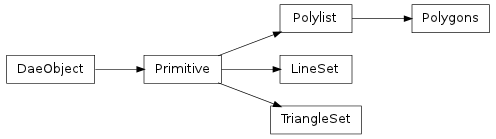#### Next topic

Class containing the data COLLADA puts in a <lines> tag, a collection of lines. The LineSet object is read-only. To modify a LineSet, create a new instance using collada.geometry.Geometry.createLineSet().

• If L is an instance of collada.lineset.LineSet, then len(L) returns the number of lines in the set. L[i] returns the ith line in the set.__init__(sources, material, index, xmlnode=None)

A LineSet should not be created manually. Instead, call the collada.geometry.Geometry.createLineSet() method after creating a geometry instance.

Methods

 __init__(sources, material, index[, xmlnode]) A LineSet should not be created manually. Instead, call the bind(matrix, materialnodebysymbol) Create a bound line set from this line set, transform and material mapping load(collada, localscope, node) save()

Attributes

 normal Read-only numpy.array of size Nx3 where N is the number of normal values in the normal_index Read-only numpy.array of size Nx3 where N is the number of vertices in the primitive. texcoord_indexset Read-only tuple of texture coordinate index arrays. texcoordset Read-only tuple of texture coordinate arrays. vertex Read-only numpy.array of size Nx3 where N is the number of vertex points in the vertex_index Read-only numpy.array of size Nx3 where N is the number of vertices in the primitive.
xmlnode

ElementTree representation of the line set.

bind(matrix, materialnodebysymbol)

Create a bound line set from this line set, transform and material mapping

getInputList()

Gets a collada.source.InputList representing the inputs from a primitive

normal

Read-only numpy.array of size Nx3 where N is the number of normal values in the primitive’s normal source array.

normal_index

Read-only numpy.array of size Nx3 where N is the number of vertices in the primitive. To get the actual normal values, one can use this array to select into the normals array, e.g. normal[normal_index].

texbinormal_indexset

Read-only tuple of texture binormal index arrays. Each value is a numpy.array of size Nx3 where N is the number of vertices in the primitive. To get the actual texture binormals, one can use the array to select into the texbinormalset array, e.g. texbinormalset[texbinormal_indexset] would select the first set of texture binormals.

texbinormalset

Read-only tuple of texture binormal arrays. Each value is a numpy.array of size Nx3 where N is the number of texture binormals in the primitive’s source array.

texcoord_indexset

Read-only tuple of texture coordinate index arrays. Each value is a numpy.array of size Nx2 where N is the number of vertices in the primitive. To get the actual texture coordinates, one can use the array to select into the texcoordset array, e.g. texcoordset[texcoord_indexset] would select the first set of texture coordinates.

texcoordset

Read-only tuple of texture coordinate arrays. Each value is a numpy.array of size Nx2 where N is the number of texture coordinates in the primitive’s source array.

textangent_indexset

Read-only tuple of texture tangent index arrays. Each value is a numpy.array of size Nx3 where N is the number of vertices in the primitive. To get the actual texture tangents, one can use the array to select into the textangentset array, e.g. textangentset[textangent_indexset] would select the first set of texture tangents.

textangentset

Read-only tuple of texture tangent arrays. Each value is a numpy.array of size Nx3 where N is the number of texture tangents in the primitive’s source array.

vertex

Read-only numpy.array of size Nx3 where N is the number of vertex points in the primitive’s vertex source array.

vertex_index

Read-only numpy.array of size Nx3 where N is the number of vertices in the primitive. To get the actual vertex points, one can use this array to select into the vertex array, e.g. vertex[vertex_index].